Next: Electromagnetic Radiation Up: Time-Dependent Perturbation Theory Previous: Perturbation Expansion

# Harmonic Perturbations

Consider a (Hermitian) perturbation which oscillates sinusoidally in time. This is usually termed a harmonic perturbation. Such a perturbation takes the form(1067)

whereis, in general, a function of position, momentum, and spin operators.

It follows from Eqs. (1064) and (1067) that, to first-order,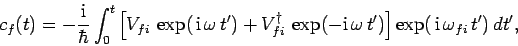(1068)

where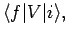(1069)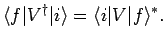(1070)

Integration with respect to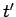yields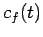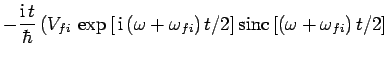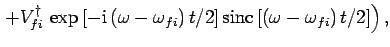(1071)

where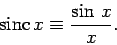(1072)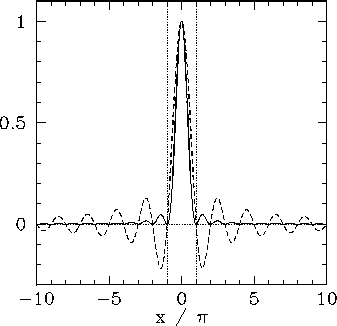Now, the functiontakes its largest values when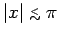, and is fairly negligible when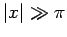(see Fig. 25). Thus, the first and second terms on the right-hand side of Eq. (1071) are only non-negligible when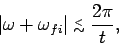(1073)

and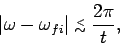(1074)

respectively. Clearly, asincreases, the ranges inover which these two terms are non-negligible gradually shrink in size. Eventually, when, these two ranges become strongly non-overlapping. Hence, in this limit,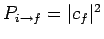yields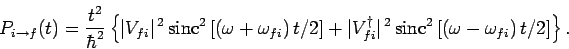(1075)

Now, the functionis very strongly peaked at, and is completely negligible for(see Fig. 25). It follows that the above expression exhibits a resonant response to the applied perturbation at the frequencies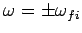. Moreover, the widths of these resonances decease linearly as time increases. At each of the resonances (i.e., at), the transition probabilityvaries as[since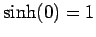]. This behaviour is entirely consistent with our earlier result (1044), for the two-state system, in the limit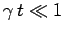(recall that our perturbative solution is only valid as long as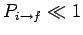).

The resonance atcorresponds to(1076)

This implies that the system loses energyto the perturbing field, whilst making a transition to a final state whose energy is less than the initial state by. This process is known as stimulated emission. The resonance at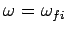corresponds to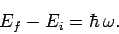(1077)

This implies that the system gains energyfrom the perturbing field, whilst making a transition to a final state whose energy is greater than that of the initial state by. This process is known as absorption.

Stimulated emission and absorption are mutually exclusive processes, since the first requires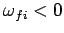, whereas the second requires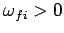. Hence, we can write the transition probabilities for both processes separately. Thus, from (1075), the transition probability for stimulated emission is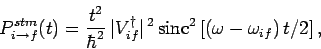(1078)

where we have made use of the facts that, and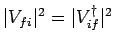. Likewise, the transition probability for absorption is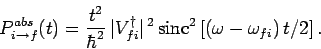(1079)Next: Electromagnetic Radiation Up: Time-Dependent Perturbation Theory Previous: Perturbation Expansion
Richard Fitzpatrick 2010-07-20